# Inception V1 Trained onExtended Salient Object Subitizing Data

Count the number of prominent items in an image

Released in 2016, this neural net is a fine-tuning of the Inception V1 model trained on the Extended Salient Object Subitizing dataset. In order to improve the performance, the dataset has been enlarged by more than a factor of 2 with synthetic images, obtained by placing segmented objects over background images.

Number of layers: 147 | Parameter count: 5,978,677 | Trained size: 24 MB |

## Examples

### Resource retrieval

Get the pre-trained net:

 In:=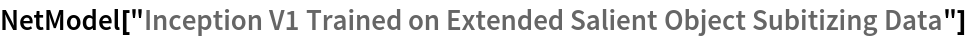Out=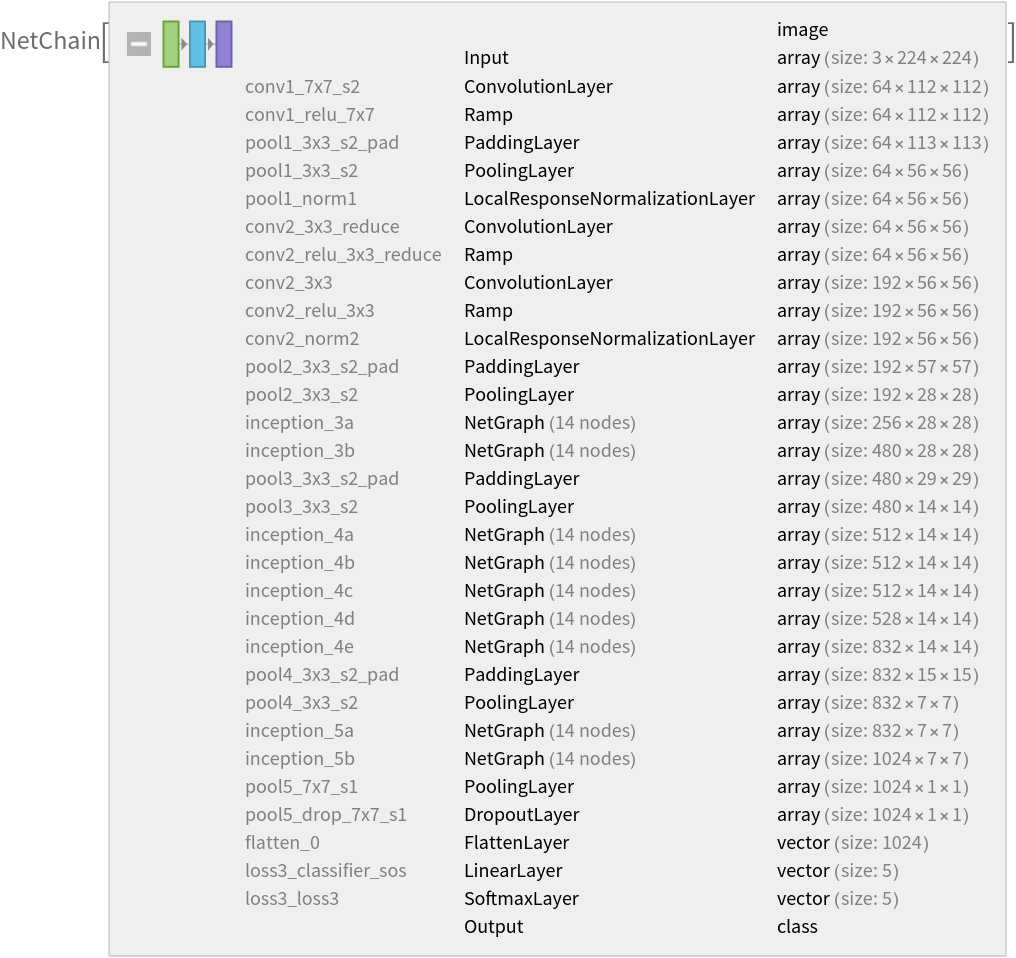### Basic usage

Obtain the number of salient objects in an image:

 In:=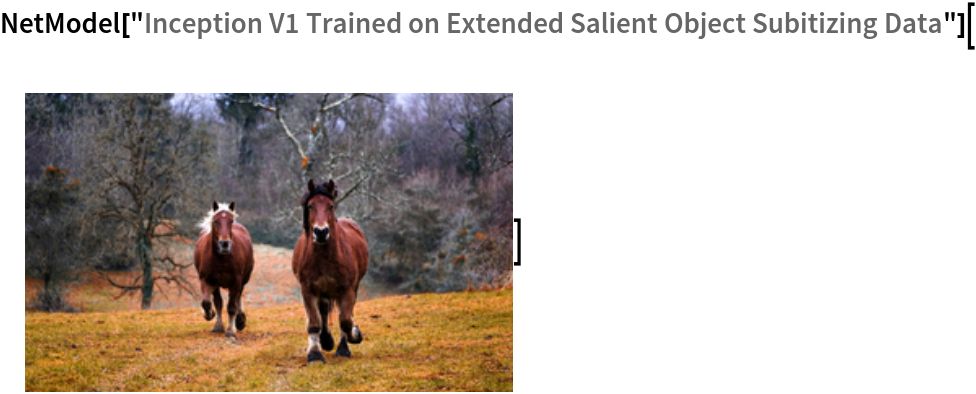Out=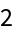Obtain the probabilities:

 In:=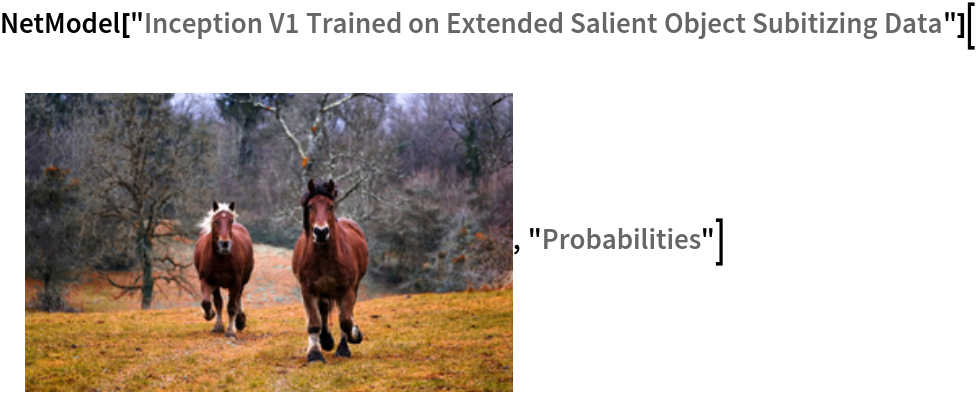Out=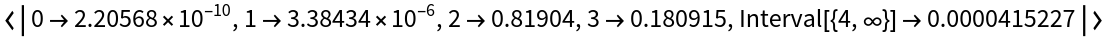### Net information

Inspect the number of parameters of all arrays in the net:

 In:=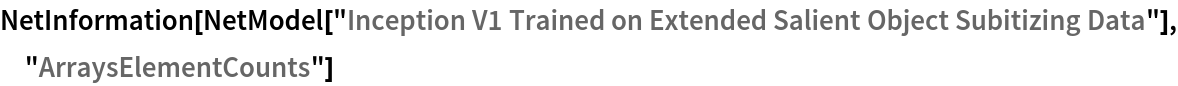Out=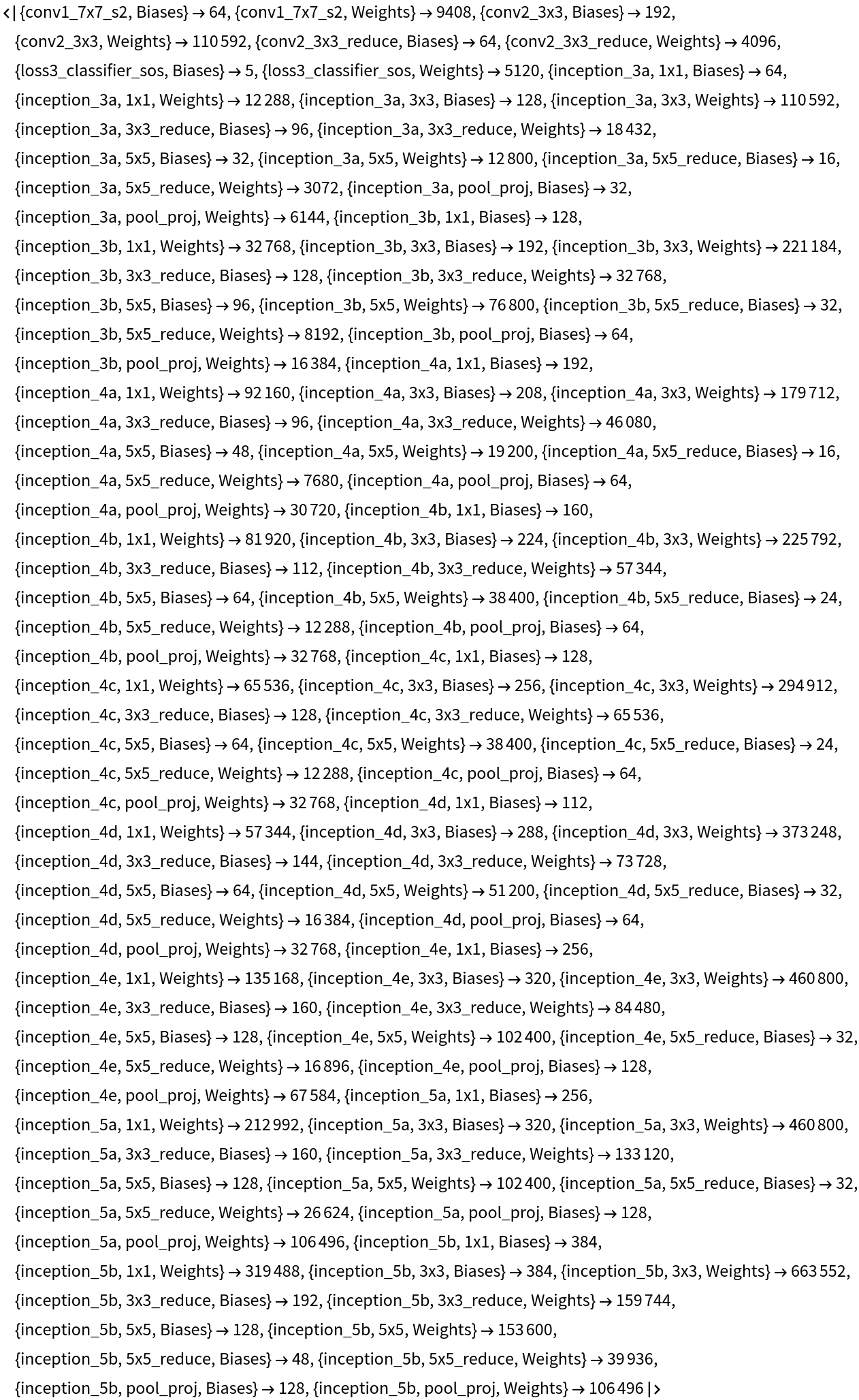Obtain the total number of parameters:

 In:=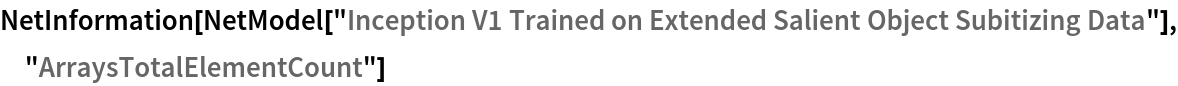Out=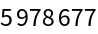Obtain the layer type counts:

 In:=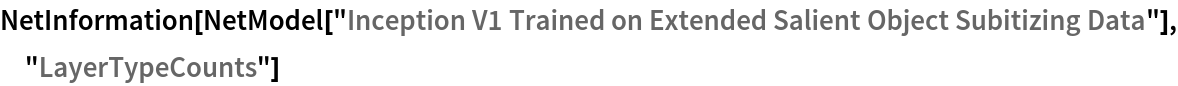Out=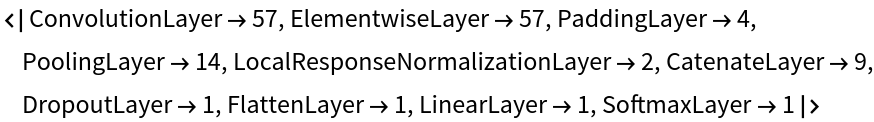Display the summary graphic:

 In:=Out=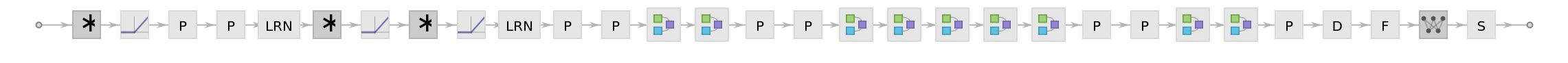### Export to MXNet

Export the net into a format that can be opened in MXNet:

 In:=Out=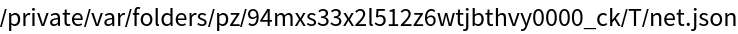Export also creates a net.params file containing parameters:

 In:=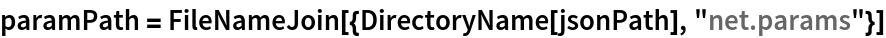Out=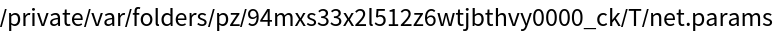Get the size of the parameter file:

 In:=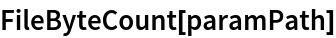Out=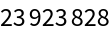The size is similar to the byte count of the resource object:

 In:=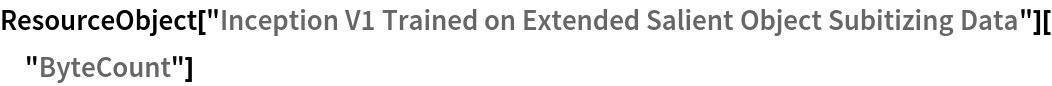Out=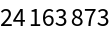Represent the MXNet net as a graph:

 In:=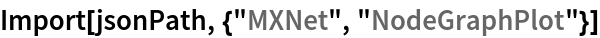Out=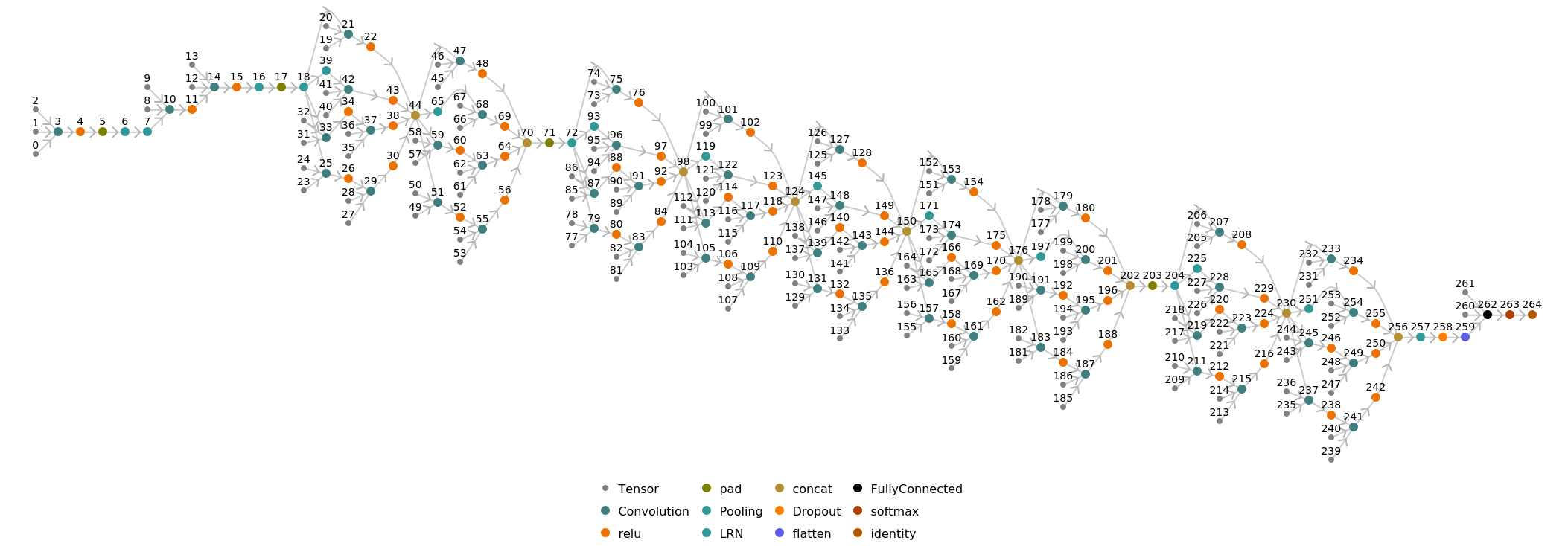## Requirements

Wolfram Language 11.2 (September 2017) or above